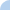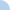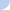Honor Geometry: Extra (optional) practice on writing equations to solve for x.

In each of the following situations, you will be given angles and angle measures. Answer the question by using either Angle Addition Property or definition of angle bisector.Quiz Log In